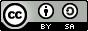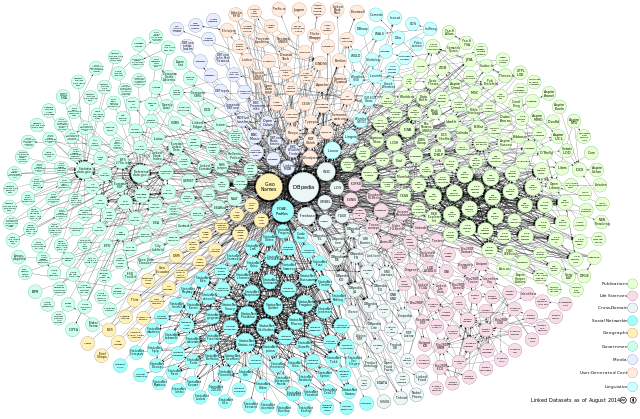# Data Mining

John Samuel
CPE Lyon

Year: 2018-2019
Email: john(dot)samuel(at)cpe(dot)fr# Goals

1. Artifical Neural Networks
2. Deep Learning
3. Reinforcement Learning
4. Data Licences, Ethics and Privacy

# 1. Artificial Neural Networks

• Inspired by biological neural networks
• Collection of connected nodes called artificial neurons.
• Artificial neurons can transmit signal from one to another (like in a synapse).
• Signal between artificial neurons is a real number
• The output of a neuron is the sum of weighted inputs.Artificial neural networks

# Perceptron

• Algorithm for supervised learning of binary classifiers
• Binary classifierArtificial neural networks

# Perceptron: Formal definition

• Let y = f(z) be output of perceptron for an input vector z
• Let N be the number of training examples
• Let X be the input feature space
• Let {(x1, d1),...,(xN, dN)} be the N training examples, where
• xi is the feature vector of ith training example.
• di is the desired output value.
• xj,i be the ith feature of jth training example.
• xj,0 = 1Artificial neural networks

# Perceptron: Formal definition

• Weights are represented in the following manner:
• wi is the ith value of weight vector.
• wi(t) is the ith value of weight vector at a given time t.Artificial neural networks

# Perceptron: Steps

1. Initialize weights and threshold
2. For each example (xj, dj) in training set
• Calculate the weight: yj(t)=f[w(t).xj]
• Update the weights: wi(t + 1) = wi(t) + (dj-yj(t))xj,i
3. Repeat step 2 until the iteration error 1/s (Σ |dj - yj(t)|) is less than user-specified threshold.

# Backpropagation

• Backward propagation of errors
• Adjust the weight of neurons by calculating the gradient of the loss function
• Error is calculated and propagated back to the network layers

# Deep neural networks

• Multiple hidden layers between the input and output layers

# Applications

• Computer vision
• Speech recognition
• Drug design
• Natural language processing
• Machine translation

# Convolutional deep neural networks

• Analysis of images
• Inspired by neurons in the virtual cortex
• Network learns the filters

# 3. Reinforcement Learning

• Inspired by behaviourist psychology
• Actions to be taken in order to maximize the cumulative award.

# 4. Data Licences, Ethics and Privacy

• Data usage licences
• Confidentiality and Privacy
• Ethics

• Volume
• Variety
• Velocity
• Veracity
• Value

# 4. Data Licences, Ethics and PrivacyPrivacy

# 4. Data Licences, Ethics and PrivacyOpen Data

# 4. Data Licences, Ethics and PrivacyLinked Open data cloud

# 4. Data Licences, Ethics and PrivacyArchived data